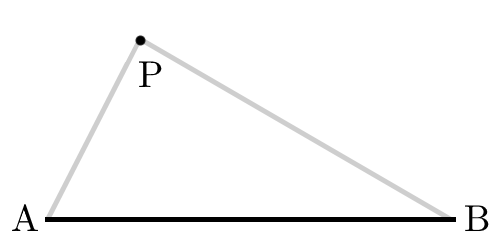# Line And Locus

Geometry Level 1A line $AB$ of length $l$ makes up one side of a triangle. The locus of all points $P$ such that $\triangle ABP$ is a right triangle with $\angle P = 90^{\circ}$ encloses an area of $\text{\_\_\_\_\_\_\_\_\_\_}$.

×